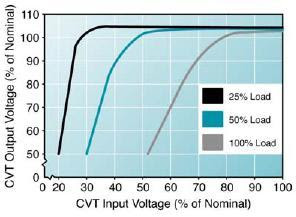## Tuesday, April 12, 2011

Constant Voltage Transformer (CVT) Sizing is anchored upon two load characteristics, both of which are related to current and must be correctly determined: the steady-state and inrush currents. If a constant voltage transformer is sized without considering the steady-state and inrush currents of all connected loads, its output voltage may sag that could affect sensitive equipment to malfunction.

A ferroresonant transformer’s ability to regulate output voltage is related to its loading percentage. The smaller the ratio between the rated current of the constant voltage transformer and the actual current drawn by the connected loads, the more efficient the CVT can regulate its output voltage. As an example, a 5 kVA ferroresonant transformer loaded to 5 kVA will not mitigate voltage sags nearly as well as the same constant voltage transformer loaded to only 2.5 kVA. In addition, the performance is even better if the same 5 kVA ferro is only loaded to 1.5 kVA. This characteristic is an important consideration for the sizing of CVTs for superior voltage regulation, as well as voltage sag mitigation.Constant Voltage Transformer Voltage Regulation According to its Loading Percentage

Sizing the Constant Voltage Transformer (CVT):

Identify the circuit connections of each load to be connected to the ferroresonant transformer.

2.     Determine the Maximum Steady-State Current

Refer to the equipment nameplate data, manufacturer’s manual or test reports. In the absence of these documents, a true RMS ammeter can be used to measure the maximum current drawn by each of the connected loads. Add the measured steady-state currents to get the total demand on the constant voltage transformer.

Caution! Make sure that all loads in the circuit to be connected to the CVT are in their normal operating state before measuring the circuit current. Set the ammeter to record the maximum steady-state RMS current during a complete process cycle, in cases where in the load turns on or off automatically.

3.     Determine the Startup or Inrush Current

In the absence of nameplate and manufacturer’s equipment data, a meter capable of capturing transient peak current values should be used to measure inrush current (i.e. Power Quality Analyzers). Next, make sure that all loads to be connected to the constant voltage transformer are turned off and have been in the off position for at least one minute. Set the meter to record maximum peak AC current over a one-millisecond period (not over a 100 millisecond period). Subsequently, with the meter set in a record and hold mode, manually turn on and off each circuit or load to be  connected to the constant voltage transformer at least eight times, leaving the load off for one minute in between each measurement. This is to increase the probability of capturing the highest inrush current, which occurs near the peak of the input voltage sine wave.

4.     Calculate the Required CVT Size

First, multiply the sum of the measured steady-state currents by the circuit voltage to get the total VA rating of the circuit or loads. After which, multiply the VA of the load by 2.5 and the product is the recommended constant voltage transformer size based on the steady-state current.

Second, multiply the maximum inrush current by the circuit voltage. Then, multiply the resulting maximum inrush VA by 0.5. This is because the ferroresonant transformer size should be at least ½ of the calculated maximum inrush VA.

Subsequently, there are now two recommended CVT VA values: one based on steady-state and the other on inrush current. Compare the calculated VA values. The larger of the two VA values is the required rating of the ferroresonant transformer for superior voltage regulation, as well as voltage sag mitigation.

An example of the calculation for the required constant voltage transformer size is shown below:

For Constant Voltage Transformer VA based on steady-state current:

Total Steady-State Current = 3.75 A
Circuit Voltage = 240 V
CVT Required VA Rating = 3.75 X 240 X 2.5
CVT Required VA Rating = 2.25 kVA

For Constant Voltage Transformer VA based on inrush current:

Maximum Inrush Current = 28 A
Circuit Voltage = 240 V
CVT Required VA Rating = 28 X 240 X 0.5
CVT Required VA Rating = 3.36 kVA

Since the VA rating based on inrush current is larger, choose that as the required transformer VA size. Thus, use 3.5 kVA CVT (next standard size).

The calculated size of the constant voltage transformer may seem rather large compared to the actual VA rating of the circuit or connected loads. Consequently, there is also the additional cost of transformer losses due to its lower loading percentage. However, the enhanced voltage regulation and sag tolerance of process control devices will likely pay for the cost of the CVT and the transformer energy losses over time through the reduced production losses, downtime and scrapped material otherwise caused by undesirable voltage variations, particularly voltage sags.

References:

Dorr, D. and Nastasi, D. (2002). Correcting Voltage Sags with Constant Voltage Transformers.
Ferraro, R., Osborne, R. and Stephens, R. (2000). The Constant Voltage Transformer (CVT) for Mitigating Effects of Voltage Sags on Industrial Equipment. Energy Systems Laboratory